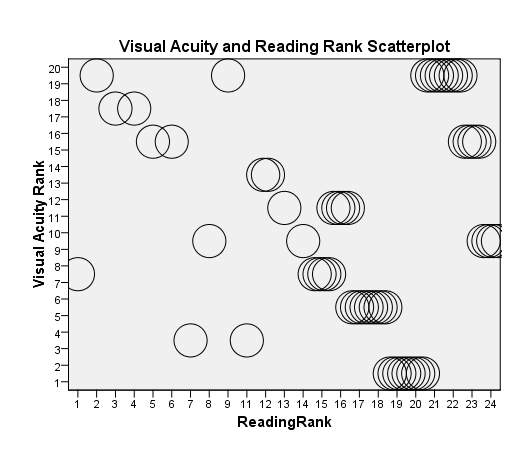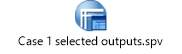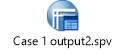# Correlation and Simple Linear Regression

10071 words (40 pages) Essay

18th May 2020 Data Analysis Reference this

Disclaimer: This work has been submitted by a university student. This is not an example of the work produced by our Essay Writing Service. You can view samples of our professional work here.

Any opinions, findings, conclusions or recommendations expressed in this material are those of the authors and do not necessarily reflect the views of UKEssays.com.

Correlation and simple linear regression methods assess the degree of strength, direction of association, and a linear summary of relationship existing between two variables, or observational units (Berg, 2004). In an effort to expose the descriptive analysis, correlational patterns resulting from the dataset DEL618_DHS618m1.sav, the writer/researcher hopes to examine the associative factors in light of the inferential statistics procedures that are paramount to the assignment. Such endeavor should help the writer/researcher to meet the goal of the theoretical basis for the assignment.

Correlation and Linear Frameworks

The correlation and linear patterns usually found in statistical analyses indicate that the role of independent and dependent variables is essential in the analysis of data as well as the levels of measurement utilized. In bivariate statistics and regression, as Berg (2004), and Myers, Gamst, and Guarino (2006) asserted, a flexibility of roles of the variables: playing one role in one context, and another role in another context can help explain their effects based on data collection methods used. This is important for the type of research design, the writer/researcher posits.

If you need assistance with writing your essay, our professional essay writing service is here to help!

A linear regression shows how a distribution is presented depending on the values of a variable x, and how another variable y varies. The relationship between these variables is the key concern. There is an effort to define a best line to ascertain the paths of the measures of central tendency (mean, variance, standard deviation…) (Berg, 2004, p.24). A simple linear equation is defined as y = a+ bx, where y is defined as the dependent variable, and x as the independent variable. The intercept, a constant, is labeled as a, and b the coefficient, is also considered as a slope. A bivariate relationship captured in a scatterplot shows how the relationship, and the shape of the bivariate between the variables are presented.

Statistical Basis

The focus of this assignment is generated from a researcher’s willingness to examine factors influencing reading scores among school children. 7 variables considered yield substantive descriptive statistics showing whether correlation relationships exist among the variables. The descriptive statistics in tables 1, 2, 3 and 4 SPSS below provide a complete picture of the variables, frequencies generated, and guided the writer/researcher description of the results. The descriptive statistics show that females have a higher frequency percentage (55.6 %) than males (24.4%). Reading rank has a higher mean than visual acuity, a lower standard deviation, and variances compared to visual acuity. This seems to suggest that the predictor and outcome variables can be considered in a bivariate domain, and correlation designs, as one variable relate to another; but there are other missing factors and further analysis to substantiate a valid conclusion or result (Keppel, Saufley, & Tokunaga, 1992).

The mean, standard deviation, median, and variances for reading rank are: 16.43 (mean), standard deviation (6.188), median (18), and variance (38.287), compared to visual acuity of  10.41 (mean), standard deviation (6.254), median (10.00) median, and 39.114(variances). For every standard deviation increase in visual acuity, there will be a 6.188 standard deviation in reading rank values.

Furthermore, for visual acuity rank, with a standard deviation of SD =6.254, skewness of .121, as depicted in table 5, and reading rank with SD =6.188, and skewness of -.965, suggesting both a skewness < 3.3 (statistics/standard error of skewness), the distribution seems to be normal. In figure 1 showing the output of a scatterplot matrix for visual acuity, and reading rank, the writer/researcher sees enough linearity due to a possible relationship between the variables when also carrying the Pearson’s correlation. However, further considerations and analysis would be needed since the scatterplot may not be too linear.

Descriptive Statistics

Table 1. Descriptive Statistics. Selected Variables

 Variables Group Mean Standard Deviation Median Frequencies (%) Variances Gender 1.56 .502 2.00 .252 Female 30 (55.6) Male 24 (44.4) Total 54 (100) ID 12.94 6.534 13.00 42.645 1 1(1.9) 2 1 (1.9) 3 1 (1.9) 4 4 (7.4) 5 1 (1.9) 6 1 (1.9) 7 4 (7.4) 8 4 (7.4) 9 4 (7.4) 10 1 (1.9) 11 1 (1.9) 12 14 (1.9) 13 4 (7.4) 14 4 (7.4) 15 4 (7.4) 16 1 (1.9) 17 1 (1.9) 18 1 (1.9) 19 4 (7.4) 20 4 (7.4) 21 1 (1.9) 22 1 (1.9) 23 1 (1.9) 24 4 (7.4) Total 54 (100) Reading in Rank 16.43 6.188 18.00 38.287 1 1 (1.9) 2 1 (1.9) 3 1 (1.9) 4 1 (1.9) 5 1 (1.9) 6 1 (1.9) 7 1 (1.9) 8 1 (1.9) 9 1 (1.9) 10 1 (1.9) 11 1 (1.9) 12 2 (3.7) 13 1 (1.9) 14 1 (1.9) 15 4 (7.4) 16 4 (7.4) 17 4 (7.4) 18 4 (7.4) 19 4 (7.4) 20 4 (7.4) 21 4 (7.4) 22 4 (7.4) 23 4 (7.4) 24 4 (7.4) Total 54 (100) Visual Acuity in Rank 10.41 6.254 10.00 39.114 1 4 (7.4) 2 4 (7.4) 3 1 (1.9) 4 1 (1.9) 5 4 (7.4) 6 4 (7.4) 7 4 (7.4) 8 1 (1.9) 9 1 (1.9) 10 5 (9.3) 11 4 (7.4) 12 1 (1.9) 13 1 (1.9) 14 1 (1.9) 15 5 (9.3) 16 1 (1.9) 17 1 (1.9) 18 1 (1.9) 19 5 (9.3) 20 5 (9.3) Total 54 (100)
 Table 2 Descriptive Statistics N Minimum Maximum Mean Std. Deviation Variance Gender 54 1 2 1.56 .502 .252 Visual Acuity Rank 54 1 20 10.41 6.254 39.114 ReadingRank 54 1 24 16.43 6.188 38.287 ID 54 1 24 12.94 6.534 42.695 Valid N (listwise) 54
 Table 3.Visual Acuity Rank Frequencies Frequency Percent Valid Percent Cumulative Percent Valid 1 4 7.4 7.4 7.4 2 4 7.4 7.4 14.8 3 1 1.9 1.9 16.7 4 1 1.9 1.9 18.5 5 4 7.4 7.4 25.9 6 4 7.4 7.4 33.3 7 4 7.4 7.4 40.7 8 1 1.9 1.9 42.6 9 1 1.9 1.9 44.4 10 5 9.3 9.3 53.7 11 4 7.4 7.4 61.1 12 1 1.9 1.9 63.0 13 1 1.9 1.9 64.8 14 1 1.9 1.9 66.7 15 5 9.3 9.3 75.9 16 1 1.9 1.9 77.8 17 1 1.9 1.9 79.6 18 1 1.9 1.9 81.5 19 5 9.3 9.3 90.7 20 5 9.3 9.3 100.0 Total 54 100.0 100.0
 Table 4. Reading Rank Frequencies Frequency Percent Valid Percent Cumulative Percent Valid 1 1 1.9 1.9 1.9 2 1 1.9 1.9 3.7 3 1 1.9 1.9 5.6 4 1 1.9 1.9 7.4 5 1 1.9 1.9 9.3 6 1 1.9 1.9 11.1 7 1 1.9 1.9 13.0 8 1 1.9 1.9 14.8 9 1 1.9 1.9 16.7 11 1 1.9 1.9 18.5 12 2 3.7 3.7 22.2 13 1 1.9 1.9 24.1 14 1 1.9 1.9 25.9 15 4 7.4 7.4 33.3 16 4 7.4 7.4 40.7 17 4 7.4 7.4 48.1 18 4 7.4 7.4 55.6 19 4 7.4 7.4 63.0 20 4 7.4 7.4 70.4 21 4 7.4 7.4 77.8 22 4 7.4 7.4 85.2 23 4 7.4 7.4 92.6 24 4 7.4 7.4 100.0 Total 54 100.0 100.0
 Table 5. Descriptive Statistics and Skewness N Minimum Maximum Mean Std. Deviation Variance Skewness Statistic Statistic Statistic Statistic Statistic Statistic Statistic Std. Error Gender 54 1 2 1.56 .502 .252 -.230 .325 Visual Acuity Rank 54 1 20 10.41 6.254 39.114 .121 .325 Reading Rank 54 1 24 16.43 6.188 38.287 -.965 .325 ID 54 1 24 12.94 6.534 42.695 .066 .325 Valid N (listwise) 54

Figure 1. Scatterplot for visual Acuity and Reading Rank MatrixBivariate Statistic and Regression

1. Multiple Regression and P-values: a) Relationship Between Social Studies, Math,

and Reading. To ascertain the relationship between these variables, the writer/researcher

posits the following research questions, and hypotheses:

RQ1: What is the relationship between social studies, math, and reading considered concurrently?

Ho: There is no statistically significant relationship between social studies, math, and reading considered concurrently.

Halt: There is a statistically significant relationship between social studies, math, and reading considered concurrently.

SPSS correlations in table 1 results reveal a correlation of .342 between social studies and math, and .647 between social studies and reading. The analysis revealed a significant and positive correlation between math and reading. r=. 342, and p= .011 for math; and r=.647, with p = .000, p < 0.01 for reading. The null hypothesis is thus rejected for the alternative hypothesis.

 Table 1. Correlations Social Studies Math Reading Social Studies Pearson Correlation 1 .342* .647** Sig. (2-tailed) .011 .000 N 54 54 54 Math Pearson Correlation .342* 1 .423** Sig. (2-tailed) .011 .001 N 54 54 54 Reading Pearson Correlation .647** .423** 1 Sig. (2-tailed) .000 .001 N 54 54 54 *. Correlation is significant at the 0.05 level (2-tailed). **. Correlation is significant at the 0.01 level (2-tailed).

b) Partial correlation coefficients for variables reading and social studies with gender held as a constant.

RQ2: What is the relationship between reading and social studies with gender held constant?

Ho: There is no statisticallysignificant relationship between reading and social studies with gender held constant.

Halt: There is a statisticallysignificant relationship between reading and social studies with gender held constant.

A partial coefficient using bivariate regression analysis was conducted for the variable reading and social studies, holding the variable gender as constant. The analysis shows a positive correlation between social studies and reading r = .643, p= < .01. With a df (51), and p value < .01(statistically significant), the null hypothesis is rejected for the alternative hypothesis. Table 2 summarizes the analysis results.

 Table 2 Partial Correlation Reading and Social Studies Control Variables Reading Social Studies Gender Reading Correlation 1.000 .643 Significance (2-tailed) . .000 df 0 51 Social Studies Correlation .643 1.000 Significance (2-tailed) .000 . df 51 0

c) Partial correlation coefficients for variables math and reading with gender held as a constant.

RQ3: Is there a relationship between math and reading with gender being held constant?

Ho: There is no statisticallysignificant relationship between math and reading with gender being held constant.

Ho: There is no statisticallysignificant relationship between math and reading with gender being held constant.

A SPSS analysis was conducted for the variables math and reading with gender held as a constant. The analysis showed a positive correlation with r =. 354, df (51), the correlation between math and reading was significant.p= .009 <0.01.

 Table 3. Partial Correlation with Math and Reading Control Variables Math Reading Gender Math Correlation 1.000 .354 Significance (2-tailed) . .009 df 0 51 Reading Correlation .354 1.000 Significance (2-tailed) .009 . df 51 0
1. Appropriate Test to Measure Correlation Between Reading in Rank, and Visual

Acuity:  A bivariatetest would be appropriate to measure the correlation between reading in rank, and visual acuity. SPSS analysis revealed a negative correlation between reading rank and visual acuity. r = -0.067, p =0.628 < 0.01 is not statistically significant.

 Table 4. Reading in Rank and Visual Acuity Correlations ReadingRank Visual Acuity Rank ReadingRank Pearson Correlation 1 -.067 Sig. (2-tailed) .628 N 54 54 Visual Acuity Rank Pearson Correlation -.067 1 Sig. (2-tailed) .628 N 54 54

Linear Regressions

1) Social Studies as a Predictor of Reading Scores: Consistent with the notion of

interactive effects of variables, espoused by Agresti (2011), linear regressions

summarizing relationships between variables ought to make sense of data, and seem to suggest more than one predictor is needed for generalizing regression analyses (Berk, 2004, p.21).

A bivariate regression analysis was conducted for social studies as a predictor for social studies. R2 =.419, F (1, 52) =37.449, reading = 55.347, Social studies = 4.257,

and p < 0.01 as statistically significant. Tables 6 to 8 summarize analysis results. Table 9 also shows the bivariate regression for social studies, and reading.

 Table 5  Variables Entered/Removeda Model Variables Entered Variables Removed Method 1 Social Studiesb . Enter a. Dependent Variable: Reading b. All requested variables entered. Table 6. Model Summary Model R R Square Adjusted R Square Std. Error of the Estimate Change Statistics R Square Change F Change df1 df2 Sig. F Change 1 .647a .419 .407 11.113 .419 37.449 1 52 .000 a. Predictors: (Constant), Social Studies Table 7ANOVAa Model Sum of Squares df Mean Square F Sig. 1 Regression 4624.798 1 4624.798 37.449 .000b Residual 6421.739 52 123.495 Total 11046.537 53 a. Dependent Variable: Reading b. Predictors: (Constant), Social Studies Table 8 Coefficientsa Model Unstandardized Coefficients Standardized Coefficients t Sig. 95.0% Confidence Interval for B Collinearity Statistics B Std. Error Beta Lower Bound Upper Bound Tolerance VIF 1 (Constant) 55.347 7.597 7.285 .000 40.102 70.591 Social Studies 4.257 .696 .647 6.120 .000 2.861 5.652 1.000 1.000 a. Dependent Variable: Reading

Table 9 Bivariate Regression for Social Studies as Predictor of Reading

Regression Weights

Variables                                            b                   B

Social studies                                   4.257           .647

R2                         .419

F              37.449

2) Math as a Predictor of Reading Scores: A bivariate regression was conducted for math and reading scores. Tables 11, 12, and 13 summarized analysis results. The bivariate regressions regression model with social studies as a predictor shows:

R2= .179

R2= .163 (adjusted), F (1, 52) =11.326 is significantly significant p=.001, and reading=74.549+1.155(math).

Table 11 below shows, B=.423 for math; thus for every standard deviation increase in math scores there is a .423 standard deviation rise in reading scores.

Table 10. Bivariate Regression for Math as a Predictor of Reading Scores

Regression weighs

Variables                                 b                 B

Math                                   1.155            .423___________________________

 Table 11 Model Summary Model R R Square Adjusted R Square Std. Error of the Estimate Change Statistics R Square Change F Change df1 df2 Sig. F Change 1 .423a .179 .163 13.208 .179 11.326 1 52 .001 a. Predictors: (Constant), Math Table 12 ANOVAa Model Sum of Squares df Mean Square F Sig. 1 Regression 1975.717 1 1975.717 11.326 .001b Residual 9070.820 52 174.439 Total 11046.537 53 a. Dependent Variable: Reading b. Predictors: (Constant), Math Table 13 Coefficientsa Model Unstandardized Coefficients Standardized Coefficients t Sig. 95.0% Confidence Interval for B Collinearity Statistics B Std. Error Beta Lower Bound Upper Bound Tolerance VIF 1 (Constant) 74.549 8.036 9.277 .000 58.424 90.674 Math 1.155 .343 .423 3.365 .001 .466 1.844 1.000 1.000 a. Dependent Variable: Reading

The SPSS outputs are included for further review by the professor if needed.References

• Agresti, A. (2011). An introduction to categorical data analysis (2nd Ed). Hoboken, NJ: John Wiley & Sons.
• Berk, R.A. (2004). Regression analysis. A constructive critique. Thousand Oaks, CA: Sage.
• Keppel, G., Saufley, W.H., Jr., & Tokunaga, H. (1992). Introduction to design and analysis: A student’s handbook (2nd ed.). New York: W.H. Freeman.
• Meyers, L. S., Gamst, G., & Guarino, A.J. (2006). Applied multivariate research. Design and interpretation. Thousand Oaks, CA: Sage.

## Related Services

View all

### DMCA / Removal Request

If you are the original writer of this essay and no longer wish to have your work published on the UKDiss.com website then please:

Related Services

Related Lectures